Case Based Questions (MCQ)

Chapter 1 Class 10 Real Numbers
Serial order wise

## A sweet seller has 420 Bundi Laddoo and 130  badam barfis. He wants to stack them is such a  way that each stack has the same number, and they  take up the least area of the tray.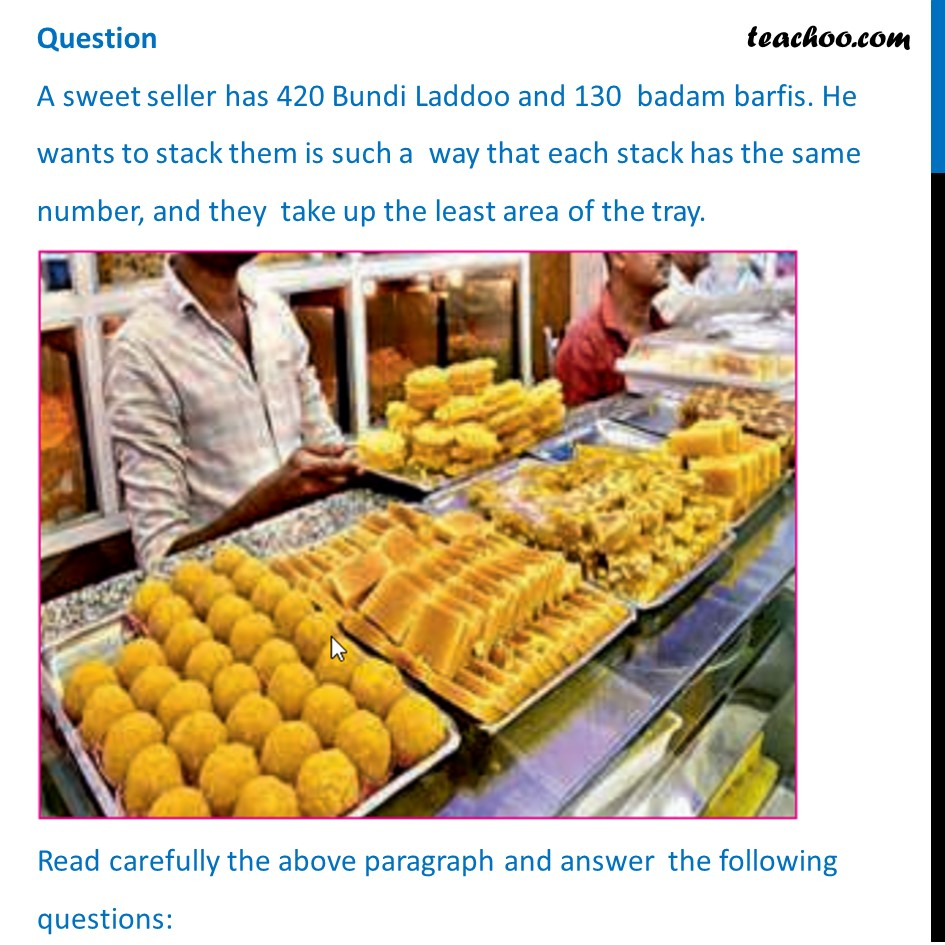## (c) 2 × 3 × 52 × 7                                      (d) 22 × 3 × 5 × 7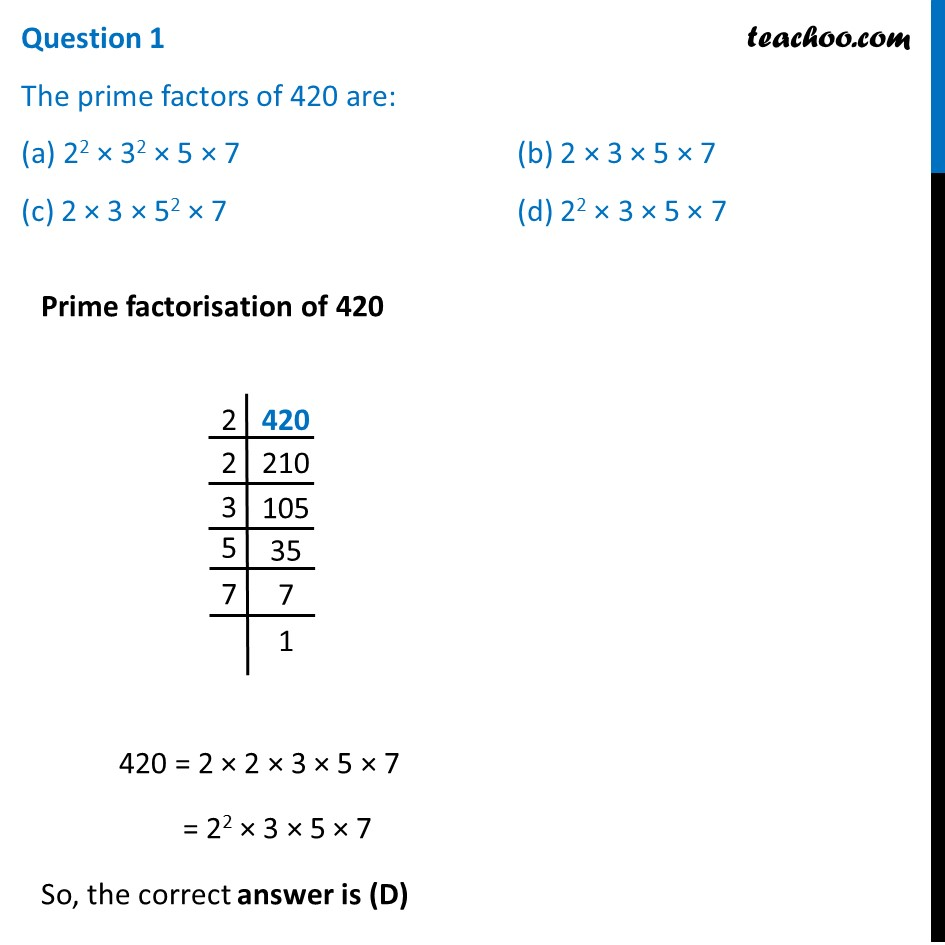## (c) 20                                                   (d) 15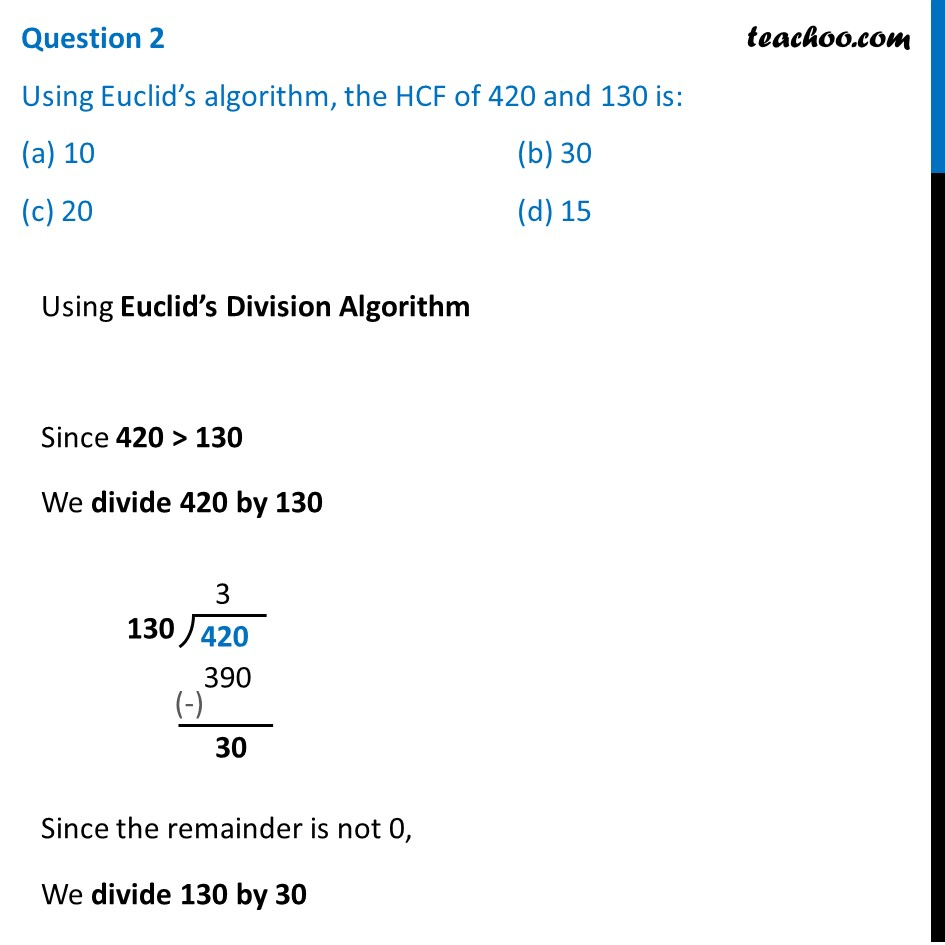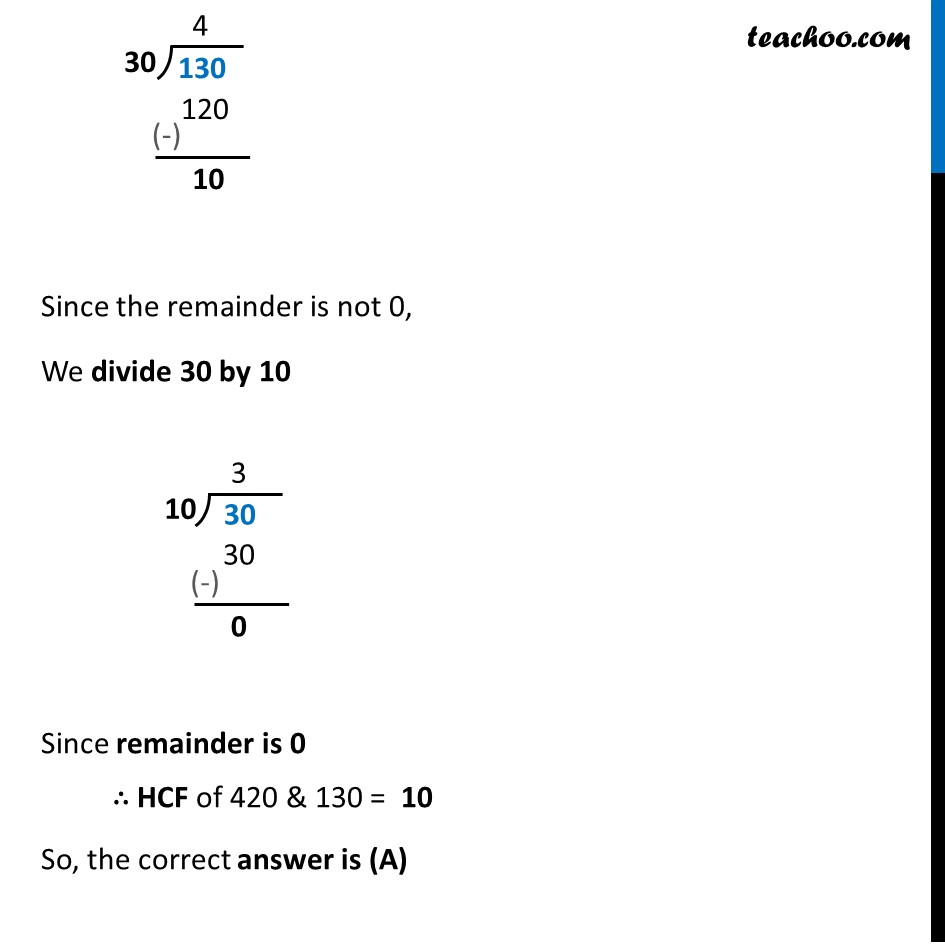## (c) 20                        (d) 15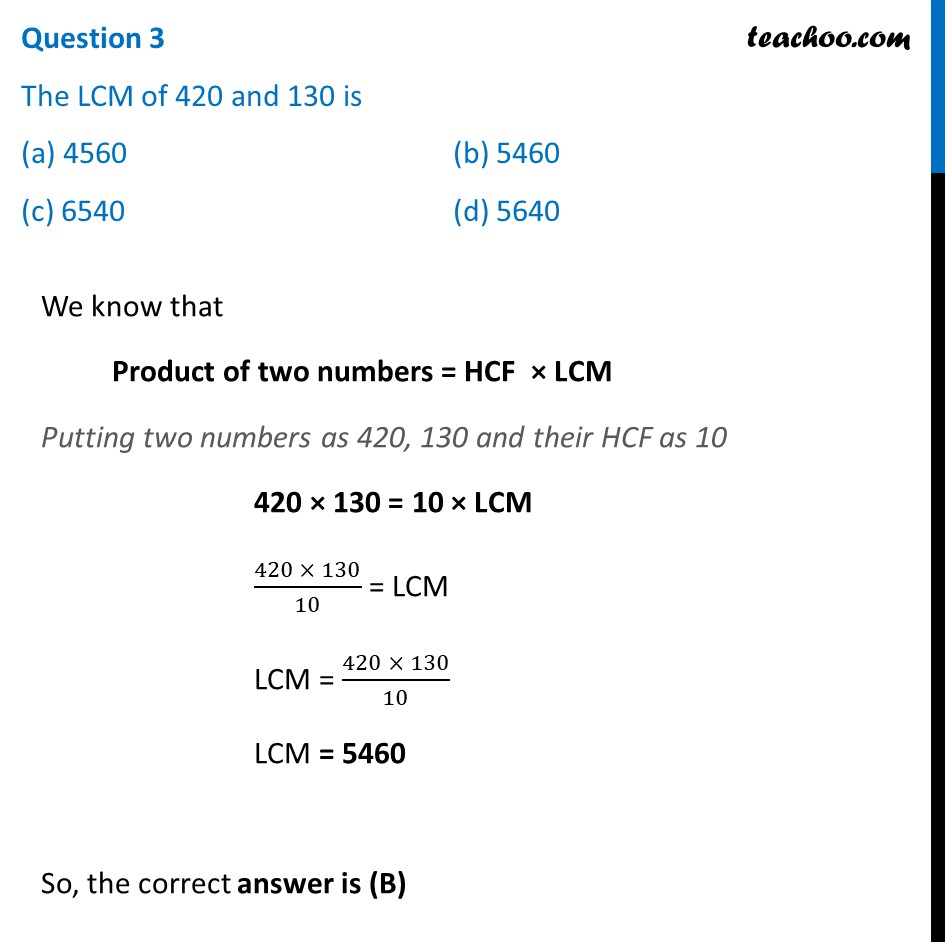## (c) 5                                                             (d) 4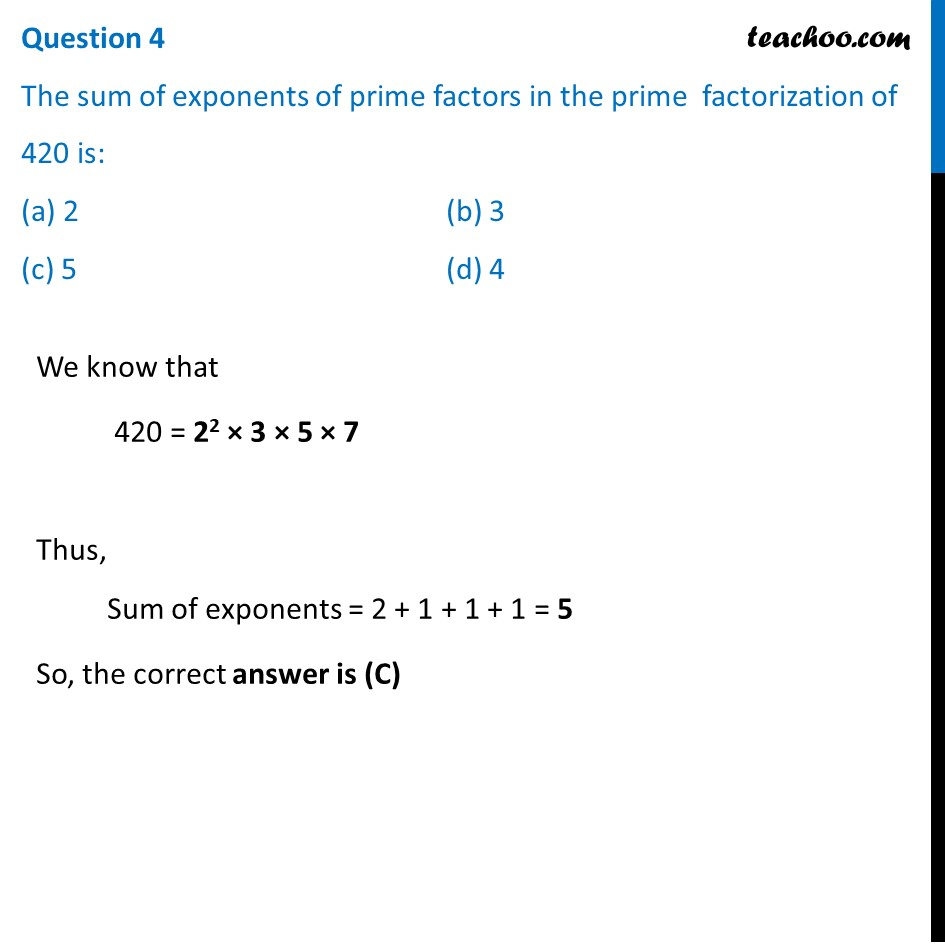## (c) 2                                                         (d) 4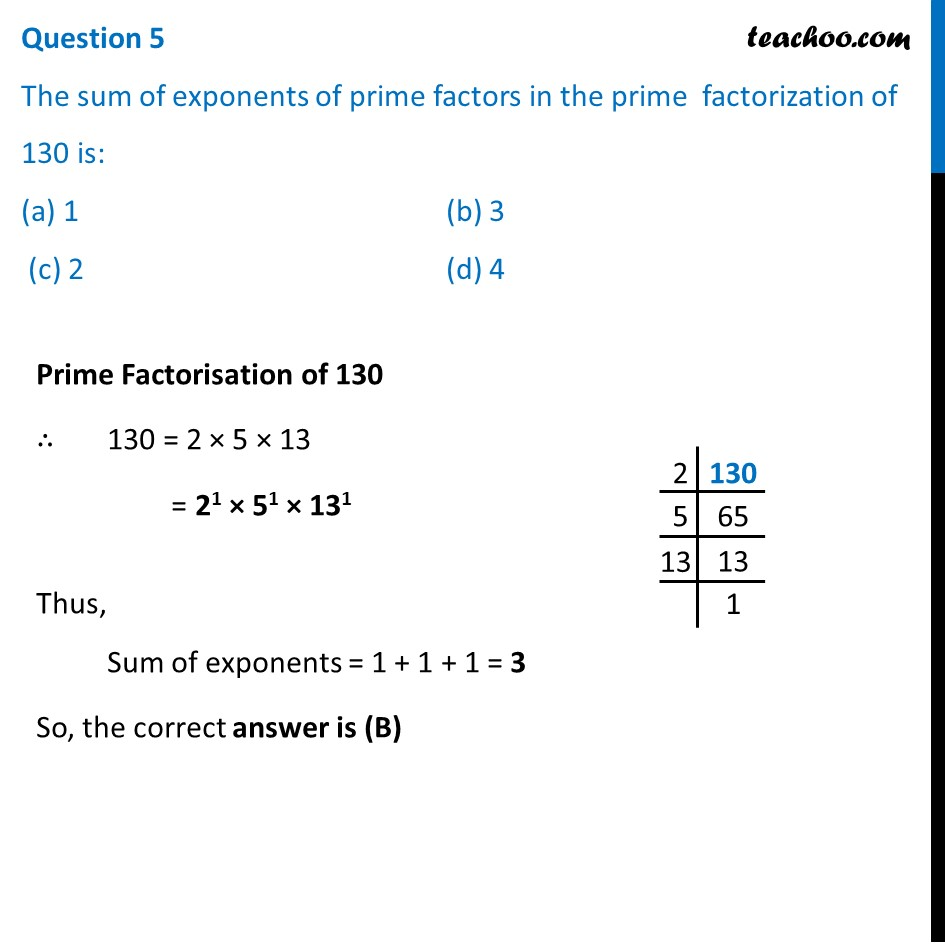Learn in your speed, with individual attention - Teachoo Maths 1-on-1 Class

### Transcript

Question A sweet seller has 420 Bundi Laddoo and 130 badam barfis. He wants to stack them is such a way that each stack has the same number, and they take up the least area of the tray. Read carefully the above paragraph and answer the following questions: Question 1 The prime factors of 420 are: (a) 22 × 32 × 5 × 7 (b) 2 × 3 × 5 × 7 (c) 2 × 3 × 52 × 7 (d) 22 × 3 × 5 × 7 Prime factorisation of 420 420 = 2 × 2 × 3 × 5 × 7 = 22 × 3 × 5 × 7 So, the correct answer is (D) Question 2 Using Euclid’s algorithm, the HCF of 420 and 130 is: (a) 10 (b) 30 (c) 20 (d) 15 Using Euclid’s Division Algorithm Since 420 > 130 We divide 420 by 130 Since the remainder is not 0, We divide 130 by 30 Since the remainder is not 0, We divide 30 by 10 Since remainder is 0 ∴ HCF of 420 & 130 = 10 So, the correct answer is (A) Question 3 The LCM of 420 and 130 is (a) 4560 (b) 5460 (c) 6540 (d) 5640 We know that Product of two numbers = HCF × LCM Putting two numbers as 420, 130 and their HCF as 10 420 × 130 = 10 × LCM (420 × 130)/10 = LCM LCM = (420 × 130)/10 LCM = 5460 So, the correct answer is (B) Question 4 The sum of exponents of prime factors in the prime factorization of 420 is: (a) 2 (b) 3 (c) 5 (d) 4 We know that 420 = 22 × 3 × 5 × 7 Thus, Sum of exponents = 2 + 1 + 1 + 1 = 5 So, the correct answer is (C) Question 5 The sum of exponents of prime factors in the prime factorization of 130 is: (a) 1 (b) 3 (c) 2 (d) 4 Prime Factorisation of 130 ∴ 130 = 2 × 5 × 13 = 21 × 51 × 131 Thus, Sum of exponents = 1 + 1 + 1 = 3 So, the correct answer is (B)